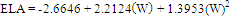### Determination of the Leaflet Area of Schinus terebinthifolius Raddi in Function of Linear Dimensions

•  Vinicius de Souza Oliveira
•  André Monzoli Covre
•  Drielly Stephania Gouvea
•  Luciano Canal
•  Karina Tiemi Hassuda dos Santos
•  Jéssica Sayuri Hassuda Santos
•  Gleyce Pereira Santos
•  Ana Paula Braido Pinheiro
•  Omar Schmildt
•  Robson Prucoli Posse
•  Marcio Paulo Czepak
•  Sara Dousseau Arantes
•  Rodrigo Sobreira Alexandre
•  José Augusto Teixeira do Amaral
•  Edney Leandro da Vitória
•  Edilson Romais Schmildt

#### Abstract

The objective of this study was to select mathematical equations that best fit the estimation of the leaf area of pink pepper (Schinus terebinthifolius Raddi) from the linear leaflet dimensions. 500 leaflets with different physiological ages of a commercial plantation were collected, located in the region of Gameleira, municipality of São Mateus, North of the State of Espírito Santo, Brazil. Was measured the length (L) along the main midrib, the largest width (W) and the observed leaf area (OLA) of each sheet. The product of the multiplication between L and W of the leaflets (LW) was determined. For the modeling the measurements of 400 leaflets were used, where OLA was used in function of L, W or LW. Based on the models found, we obtained the estimated leaf area (ELA). A simple linear regression was fitted for each proposed model of OLA in function of ELA. We tested the hypotheses H0: β0 = 0 versus Ha: β0 ≠ 0 and H0: β1 = 1 versus Ha: β1 ≠ 1, using Student’s t test at 5% probability. The mean values of ELA and OLA were compared by Student’s t test 5% probability. It was determined the mean error (E), mean absolute error (MAE), root mean square error (RMSE) and Willmott d index. The best adjusted equation was chosen by linear coefficient (β0) not different from zero, angular coefficient (β1) not unlike one, non-significant values of ELA and OLA, E, EAM and RQME closer to zero and Willmott’s index d closer to one. In this way, the leaf area of leaflets of Schinus terebinthifolius Raddi can be estimated by the quadratic model equation ELA = -2.6646 + 2.2124W + 1.3953(W)2, using only the width of the leaves as a measure.This work is licensed under a Creative Commons Attribution 4.0 License.
• ISSN(Print): 1916-9752
• ISSN(Online): 1916-9760
• Started: 2009
• Frequency: monthly

### Journal Metrics

(The data was calculated based on Google Scholar Citations)

• Google-based Impact Factor (2018): 2.28
• h-index (December 2018): 31
• i10-index (December 2018): 304
• h5-index (December 2018): 22
• h5-median (December 2018): 27